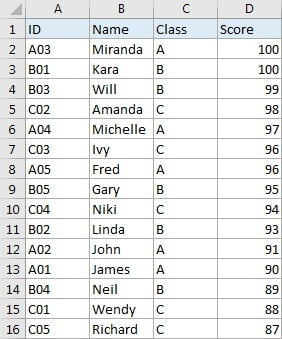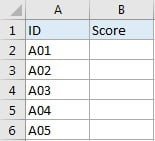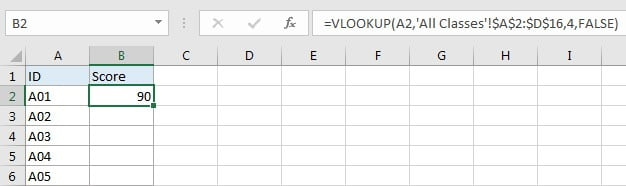# VLOOKUP – Retrieve Data from Another Worksheet

VLOOKUP is one of the key functions among all lookup & reference functions in Excel. It can scan and retrieve data from a static or dynamic table based on your lookup value. It can perform approximate match or exact match by setting lookup matching modes.

Today, we will show you the way to apply VLOOKUP  function to retrieve data from lookup table on another worksheet. It is similar to applying VLOOKUP  formula to retrieve data on the same worksheet. In our daily work, we often need to extract data from another worksheet using VLOOKUP function, if this is a confusing question for you, today’s article will help you to solve your problem.

## EXAMPLETable1: This table is located in sheet “All Classes”.Table2: This table is located in sheet “Class A”. Table1 and table2 are in the same workbook.

In this example, we want to get score from “Score” column of table1 in sheet “All Classes” to fill “Score” column of table2 in sheet “Class A” correspondingly.

We can see that table1 is a summary and all IDs from table2 can be found in this table. Besides, they both list ID as the first column. So, we can apply VLOOKUP function to perform an exact match lookup to get score for each student properly.

## ANALYSIS

This is a typical example that can be solved by VLOOKUP function. Unlike basic VLOOKUP examples, in this instance, for class A, ID and scores are not displayed in the same table, scores are only listed in the last column of table on another worksheet, and this table is our lookup table. To retrieve data from lookup table on another worksheet, we just need to add ‘sheet name’! before range reference.

Actually, when typing VLOOKUP the second argument “table_array”, you can directly move to the worksheet you can find your lookup table (then this worksheet is displayed on top), drag and drop the lookup table, then ‘sheet name’! with range reference is entered automatically and shown in the formula bar properly.

We create below formula with VLOOKUP for this instance:

`=VLOOKUP(A2,'All Classes'!\$A\$2:\$D\$16,4,FALSE)`

## FORMULA

Input formula =VLOOKUP(A2,’All Classes’!\$A\$2:\$D\$16,4,FALSE) into B2, press ENTER, verify that score  “90” is returned by formula properly for ID A01.## FUNCTION INTRODUCTION

a. VLOOKUP function looks up the input value from the first column of the given table, and returns the value we want from a particular position (row number depends on the row of lookup value, column number depends on the input argument col_index_num).

Syntax:

`=VLOOKUP(lookup_value, table_array, col_index_num,[range_lookup])`

For argument “range_lookup”, there are two matching modes “True” and “False”.

• The default mode is “True”, so if this optional argument is omitted, this value is set to “True” automatically.
• If we set “range_lookup” to “True”, VLOOKUP function performs an approximate match.
• If we set “range_lookup” to “False”, VLOOKUP function looks up data restrictively by performing an exact match. If look up value is not found in table, formula returns error “#N/A”.

## EXPLANATION

`=VLOOKUP(A2,'All Classes'!\$A\$2:\$D\$16,4,FALSE)`

lookup_value is input into A2 (ID “A01”)

table_array is ‘All Classes’!\$A\$2:\$D\$16

col_index_num is 4. Because scores are saved in the fourth column of this table.

range_lookup is set to FALSE. VLOOKUP function performs an EXACT match.

a. In this formula, the look up value is “A01”, VLOOKUP scans this value from the first column of table in worksheet “All Classes”. It continues scanning until it meets the value that is exactly the same with lookup value. In this case, VLOOKUP function will stop scanning when meeting “A01” and stay in current row.

b. Table array is ‘All Classes’!\$A\$2:\$D\$16, this table contains lookup value in its first column and expected value in its last column. We add “\$” before row and column to lock this range, so when copying down the formula, the range doesn’t change. Besides, as we mentioned before, sheet name is added before range reference, so VLOOKUP function can find out lookup table from proper worksheet.

c. As Score column is the fourth column in lookup table, so we enter 4 as col_index_num. VLOOKUP function retrieves data at the intersection of column “Score” and row “A01”.

d. We set range_lookup mode to “FALSE”, so VLOOKUP function performs an exact match lookup and this operation can make sure that VLOOKUP returns correct score for each student. If lookup ID cannot be found in the given table, an error #N/A will be returned.

### Related Functions

• Excel INDEX function
The Excel INDEX function returns a value from a table based on the index (row number and column number)The INDEX function is a build-in function in Microsoft Excel and it is categorized as a Lookup and Reference Function.The syntax of the INDEX function is as below:= INDEX (array, row_num,[column_num])…
• Excel VLOOKUP function
The Excel VLOOKUP function lookup a value in the first column of the table and return the value in the same row based on index_num position.The syntax of the VLOOKUP function is as below:= VLOOKUP (lookup_value, table_array, column_index_num,[range_lookup])….
Related Posts

Basic Price Discount Calculation with Excel VLOOKUP Function

We often encounter product discounts in our shopping. Depending on the level of spending, the mall will offer different percentages of discounts. Usually, the more you spend, the bigger the discount, while the less you spend, the smaller the discount. ...

Calculate Grades With VLookup in Excel

Why Should You Calculate Grades With VLookup Excel? If you're looking for a simple way to find out a student's grades, VLOOKUP Excel can do the trick. This function uses a lookup table to find the values and sort them ...

Convert State Names To Abbreviations

Assume that you got a task to convert the full state's name into the abbreviations in MS Excel, and for doing this task, you might choose to do it manually, which is an acceptable way only if you don't have ...

Find and Replace Multiple Values

This post will guide you how to find and replace multiple values at once with VBA macro or using formula  in Excel. How do I make multiple find and replace in Excel. Suppose that you have a few cells containing ...

Excel XLOOKUP Function

Excel XLOOKUP Function was added into Excel as a beta feature in August 2019 and is now accessible exclusively in Microsoft 365. (as of July 2021). However, if you fall into this category and often deal with big sets of ...

VLOOKUP Formula | Faster Trick with 2 VLOOKUPS

This post will guide you how to use 2 VLOOKUPS function to looking up data entries from a given range of cells in Microsoft Excel. VLOOKUP with 2 lookups can be faster than a single VLOOKUP in certain scenarios. The ...

VBA Macro For VLOOKUP From Another Sheet

In the previous post, you should know that how to fix or remove the #N/A error when using VLOOKUP formula to lookup value from another sheet. And this post will show you how to use VBA code to vlookup data ...

VLOOKUP From Another Sheet Not Working

In the previous post, you should know that how to fix or remove the #N/A error when using VLOOKUP formula to lookup value from another sheet. And this post will show you reasons why your VLOOKUP formula is not working ...

Fix #N/A Error For VLOOKUP From Another Sheet

This post will show you how to fix the #N/A error why it occurs when you extract values from another sheet using VLOOKUP function in Excel 2016,2013,2010 or other Excel versions. How can you correct a #N/A error in VLOOKUP ...

Get Employee Information by VLOOKUP

VLOOKUP is one of the key functions among all lookup & reference functions in Excel. Today, in this article, we will show you the way to apply VLOOKUP to retrieve employee information. I hope this article will help you in ...

Sidebar